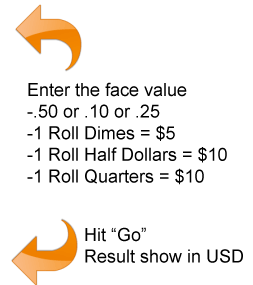# Silver Coin Calculator

Face Value Silver Value Calculator

Enter the face value of bag of coins, a roll of coins, or even an individual coin into the silver coin calculator to get the total melt value. It's based off of a 90% silver per coin esitmation, and the current spot price of silver 23.65 As a quick rule of thumb, if a coin was produced before 1965 it is 90% silver. (Dimes, Quarters, Half Dollars only). Or get the current prices for junk silver bags.\$

If you are looking for the silver content value of us coins then use this link to get there.

### How is Melt Value Calculated?

For the sake of simplicity the companies usually figure up values based on on coin face values x the value of a single dollar.

So the current value of \$1 face is \$17.1 <---- That is the live actual price.

It doesn't matter if you have 4 quarters, 10 dimes, or 2 halves. The melt value for \$1 face is all the same.

Note of Importance: Morgans and Peace Dollars do not follow this rule, they are actually worth a bit more because they have slightly more silver than \$1 face of dimes, quarters, or halves.

Use our face value calculator to figure out the value of your stack of 90% silver coins now.

*this calculates the value of your 90% coins using the same formula used to calculate the price for the junk silver prices today.